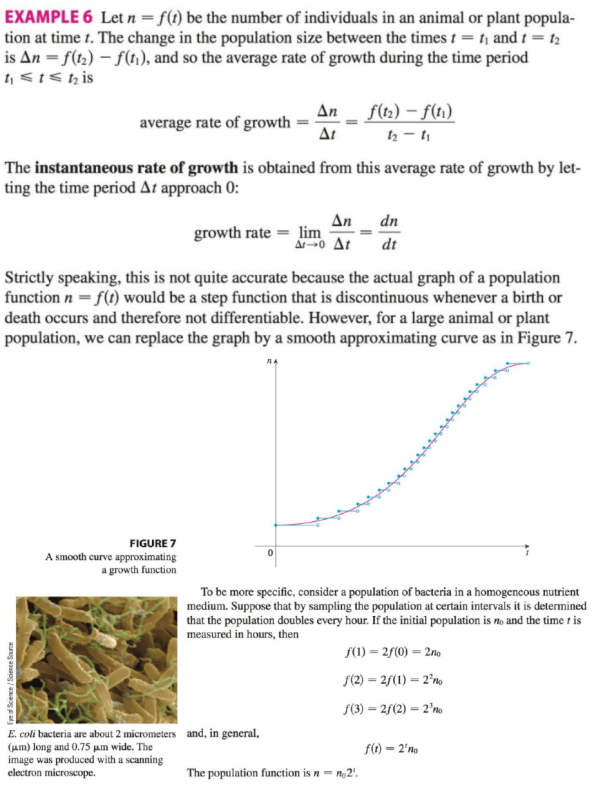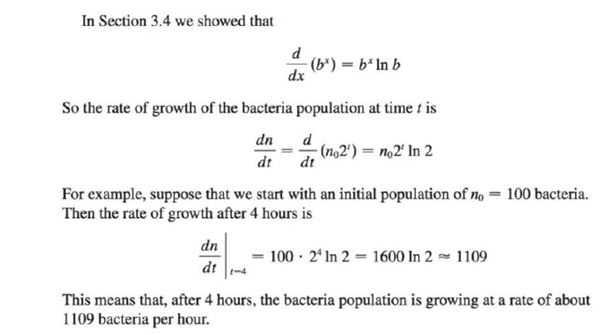Chapter 3.7, Problem 25E

Chapter
Section
Textbook Problem

In Example 6 we considered a bacteria population that doubles every hour. Suppose that another population of bacteria triples every hour and starts with 400 bacteria. Find an expression for the number n of bacteria after t hours and use it to estimate the rate of growth of the bacteria population after 2.5 hours.EXAMPLE 6To determine

To find: the expression for the number of bacteria after t, and estimate the rate of growth of the bacteria population after 2.5 hours.

Explanation

Given:

The initial population n0 is 400.

Population of the bacteria triples in one hour as per the rate expression given below.

n(t)=n0×3t (1)

Calculation:

Expression for n bacteria in t hours.

Substitute the 400 for n0 in (2).

n(t)=400×3t (2)

Determine the rate of growth of population after 2.5 hours.

Differentiate equation (2) with respect to t.

dndt=400×3t(ln3)=400×3t(1

Still sussing out bartleby?

Check out a sample textbook solution.

See a sample solution

The Solution to Your Study Problems

Bartleby provides explanations to thousands of textbook problems written by our experts, many with advanced degrees!

Get Started

Solve the equations in Exercises 112 for x (mentally, if possible). x3=1

Finite Mathematics and Applied Calculus (MindTap Course List)

In Exercises 2340, find the indicated limit. 34. limx1x3+12x3+2

Applied Calculus for the Managerial, Life, and Social Sciences: A Brief Approach

Find the centroid of the region shown. 13.

Single Variable Calculus: Early Transcendentals

True or False: The function whose graph is given at the right is a probability density function.

Study Guide for Stewart's Single Variable Calculus: Early Transcendentals, 8th